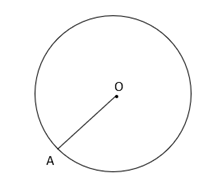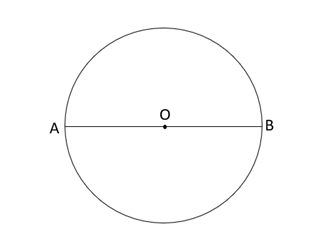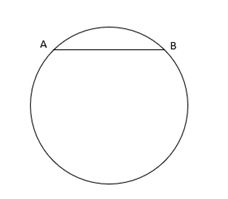# Circle | Radius | Diameter | Circumference | Chord

Circle, deals with various concepts which are as under:-

• Diameter of a circle
• Circumference of a Circle
• Chord of a Circle

A line segment having one end point on the circumference of the circle and other end point at the center of the circle is called the radius of a circle.Here, OA is the radius of the circle having point O at the center of the circle, and point A on the circumference of the circle.

### Diameter of a circle

A line segment which passes through the center of a circle, and whose end points lie on the circumference of the circle is called the Diameter of a circle.In the above figure, AB, is a line segment which passes through the center O, and its end points (A and B) lie on the circumference of the circle. Hence AB is the diameter of the given Circle.

### Circumference of a Circle

The length of the boundary of a circle is called circumference of a circle.

### Chord of a Circle

Line segment having its both the end points on the circumference of the circle is called the chord of the circle.Here, AB is the chord having its end points ( A and B ) , which lie on the circumference of the circle.